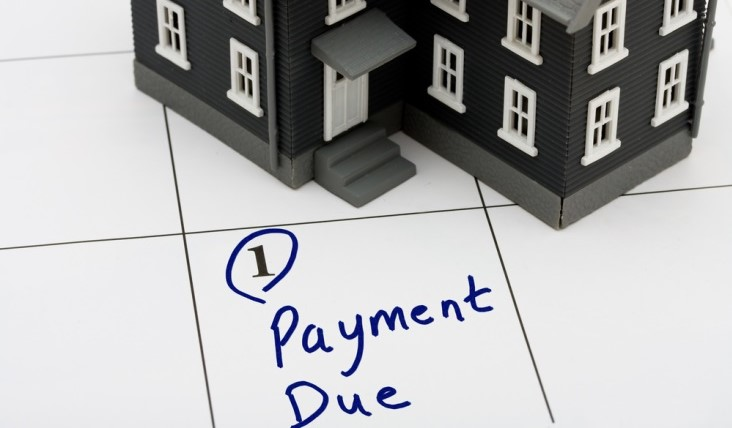Home / Money Blog

## Paying off a HELOCNote: You can use any financial calculator to do this problem, but if you want the BEST, you can get our 10bii Financial Calculator for iOS, Android, Mac, and Windows!THE SCENARIO A friend of mine has a Home Equity Line of Credit (HELOC) that she got nearly 10 years ago. For those 10 years, the loan has been interest only, and the rate is Prime minus 1%. Most HELOCs are 'adjustable', which means that their interest rate changes over time. Adjustable loans are generally tied to an 'index', such as Prime, COFI (Cost of Funds Index), or LIBOR (London Interbank Offered Rate), and generally have some spread, which is the amount above or below the index the charged rate will be. My friend's loan is tied to Prime, and the spread is -1 percentage point. So if Prime is 4% in a given month, my friend's loan will be at 3% interest for that month. This HELOC is a bit different than other loans I've run across, in that after the 10-year interest-only period, it switches to a 10-year straight-line amortization. What that means is that when the interest-only period ends, the lender takes the amount still owed, divides it by the 120 months in the 10-year payoff period, and increases the payment for each of those months by the result. Each monthly payment still has an interest component, and that's figured the same way it always has been: multiplying the monthly rate by the amount still owed when the month began. Don't worry, that's less confusing than it sounds, and it'll be clearer once we start seeing some actual numbers. Okay, my friend currently owes \$89,598.55, and her monthly (interest-only) payment is \$245.96. My questions is: If she came up with the money to pay off the loan up-front, what would her return on the \$89,598.55 be? For the purposes of this question, we'll assume that the interest portion of each payment would be static at \$245.96, even though in reality it would change each month as the principal gets paid off, and as the interest rate fluctuates over time.
THE SOLUTION This question is actually pretty straightforward, and it has 3 steps. First, we have to find out how much principal will be required on each of the 120 payments. Next, we have to find out what the principal + interest payment would be. Last, we need to find the ROI on the \$89k payment. Step 1 The principal portion of each payment will be \$89,598.55 / 120 = \$746.65. Step 2 The total monthly payment will be \$746.65 + \$245.96 = \$992.61 Step 3 First things first, make sure the calculator is using 12 Payments per Year. N: 120 I/YR: (this is what I'm trying to find) PV: -89,598.55 (the amount my friend will have to pay to satisfy the loan) PMT: 992.61 (the amount of each payment she won't have to make) FV: 0

The answer to the question is that my friend will get a 5.95% return on her \$89k if she pays off the loan immediately instead of making the monthly payments over the next 10 years.

What do you think? Would you pay off the loan on day 1? Or do you think you could do something with the \$89k that would generate more money over time, thus potentially offsetting the now-larger monthly payments? Let us know in the comments!